# Important Microeconomics Keywords

Please Share with maximum friends to support the Initiative.

 Microeconomics describes the pricing of products and money, causes of different prices to different people, how can provide more or less benefit to producers, consumers, and others, and how individuals best coordinate and cooperate. Microeconomics provides a more complete and detailed understanding than macroeconomics. In recent times UPSC has been asking Questions from this aspect,So Aspirants are advised to have a quick glance over it.

Seller is an individual, or entity, who exchanges any good or service in return for payment. In the financial markets, a seller is a person or entity who is offering security they hold to be purchased by someone else. In the options market, a seller is also called a writer. The writer is on one side of the contract and receives a premium for selling the option.

The interest rate is the amount a lender charges for the use of assets expressed as a percentage of the principal. The interest rate is typically noted on an annual basis known as the annual percentage rate (APR). The assets borrowed could include cash, consumer goods, or large assets such as a vehicle or building.

 Annual percentage rate (APR) refers to the annual rate of interest charged to borrowers and paid to investors. APR is expressed as a percentage that represents the actual yearly cost of funds over the term of a loan or income earned on an investment.

 Premium has multiple meanings, first being the total cost to buy an option. A premium is also the difference between the price paid for a fixed-income security and the security's face amount at issue. Finally, a premium is also the specified amount of payment required periodically by an insurer to provide coverage under a given insurance plan for a defined period of time.

Total variable cost is the aggregate amount of all variable costs associated with the cost of goods sold in a reporting period. It is a key component in the analysis of corporate profitability.

Average variable cost is defined as the total variable cost per unit of output.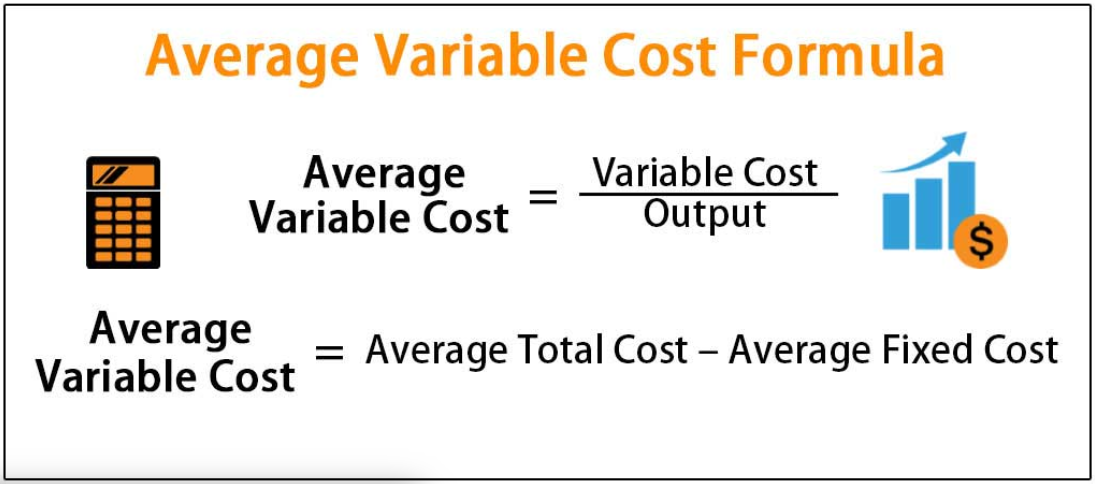The average cost is the sum of the average variable cost and average fixed cost. It is also defined as the Total cost per unit of output.

Average product is defined as output per unit of the variable input.

Average revenue is defined as total revenue per unit of output.

Barriers to entry are the economic term describing high start-up costs or other obstacles that prevent new competitors from easily entering an industry or business sector. Barriers to entry benefit existing firms because they protect their revenues and profits.

Break-even point is the point on the supply curve at which a firm earns a normal profit. In simple words, the break-even point can be defined as a point where total costs (expenses) and total sales (revenue) are equal.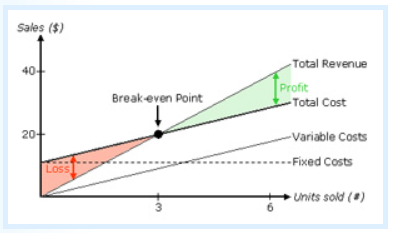The budget line is a graphical representation of all possible combinations of two goods that can be purchased with given income and prices, such that the cost of each of these combinations is equal to the money income of the consumer.

Example: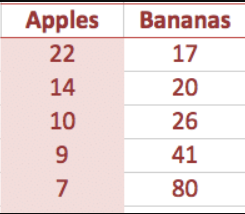A budget line shows the combination of goods that can be afforded with your current income.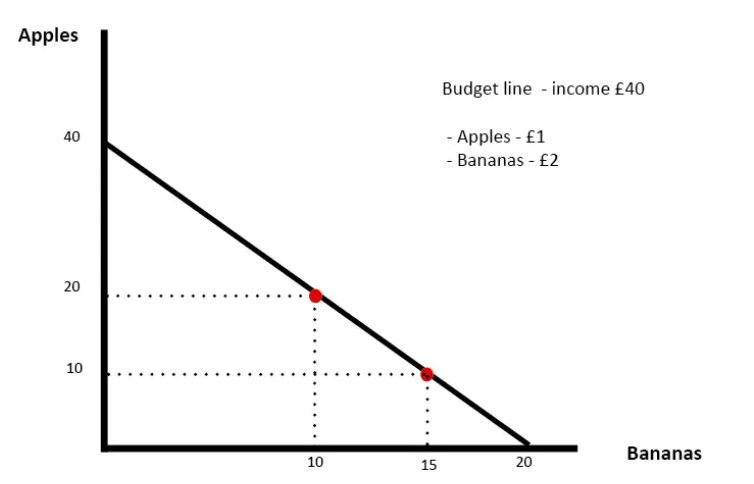Budget set is the collection of all bundles that the consumer can buy with her income at the prevailing market prices.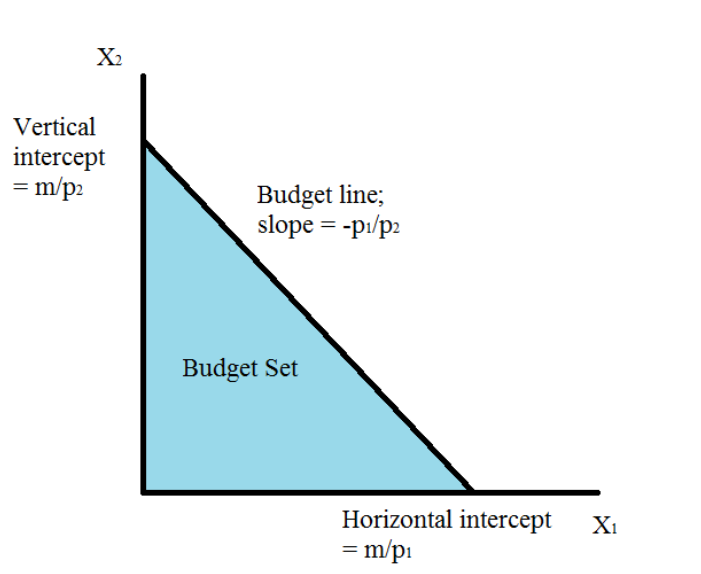Constant returns to scale is a property of production function that holds when a proportional increase in all inputs results in an increase in output by the same proportion.

Cost function describes the least cost of producing each level of output given prices of factors of production and technology.

Decreasing returns to scale is a property of production function that holds when a proportional increase in all inputs results in an increase in output by less than the proportion.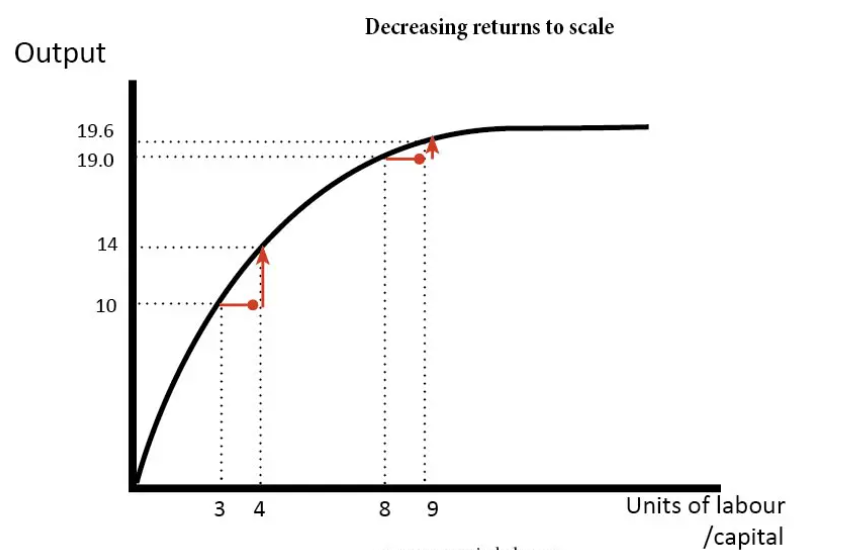Disposable income, also known as disposable personal income (DPI), is the amount of money that households have available for spending and saving after income taxes have been accounted for.

• DPI=Personal Income−Personal Income Taxes

Increasing returns to scale is a property of production function that holds when a proportional increase in all inputs results in an increase in output by more than the proportion.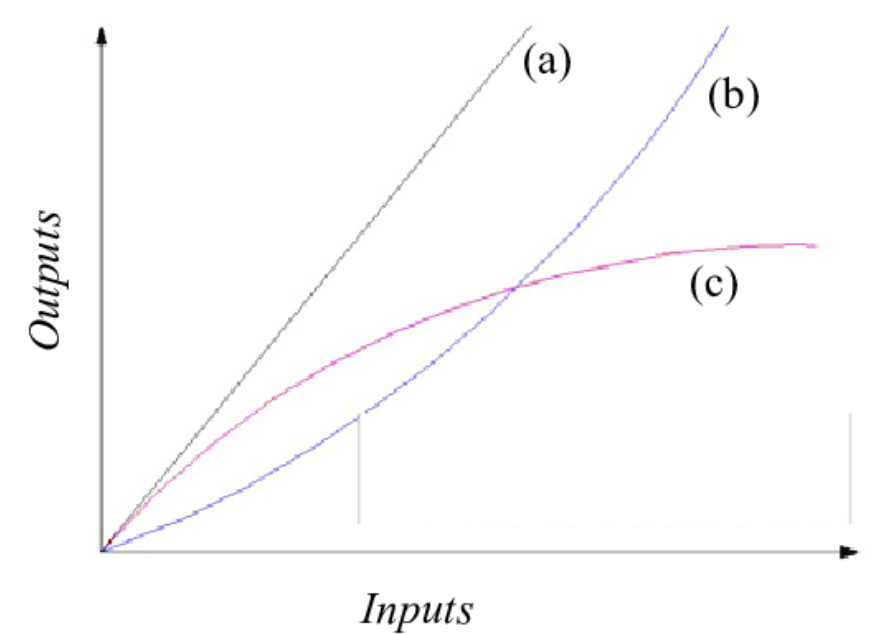The demand curve is a graphical representation of the relationship between the price of a good or service and the quantity demanded for a given period of time. In a typical representation, the price will appear on the left vertical axis, the quantity demanded on the horizontal axis.Demand function A consumer’s demand function for a good gives the amount of the good that the consumer chooses at different levels of its price when the other things remain unchanged.

Equilibrium is a situation where the plans of all consumers and firms in the market match.

Excess demand: If at a price market, demand exceeds market supply, it is said that excess demand exists in the market at that price.

Excess supply If at a price market, supply is greater than market demand, it is said that there is excess supply in the market at that price. The firm’s supply curve shows the levels of output that a profit maximising firm will choose to produce at different values of the market price.

Fixed Input: An input whose quantity cannot be changed in the time period under consideration. The relevant time period is usually termed the short run. The most common example of a fixed input is capital. The alternative to fixed input is variable input. A fixed input, such as capital, provides the “capacity” constraint for the short-run production of a firm. A variable input, such as labour, provides the means of changing short-run production. As larger quantities of a variable input are added to a fixed input, the variable input becomes less productive, which is the law of diminishing marginal returns.

Giffen goods Demand rises when the price rises and falls when the price falls.

Income effect: The income effect is the change in demand for a good or service caused by a change in a consumer's purchasing power resulting from a change in real income. This change can be the result of a rise in wages etc., or because existing income is freed up by a decrease or increase in the price of a good that money is being spent on.

Increasing returns to scale is a property of production function that holds when a proportional increase in all inputs results in an increase in output by more than the proportion.

Indexation is a system of economic regulation where wages and interests are tied to the cost of living index in order to reduce the effects of inflation.

An indifference curve is a graph showing a combination of two goods that give the consumer equal satisfaction and utility. Each point on an indifference curve indicates that a consumer is indifferent between the two and all points give him the same utility.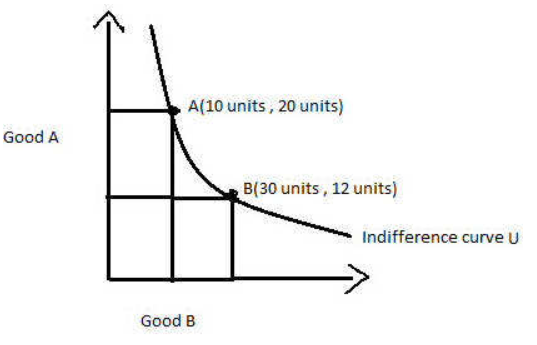Inferior good, A good for which the demand decreases with an increase in the income of the consumer is called an inferior good.

Income Elasticity of Demand measures the responsiveness of demand for a particular good to changes in consumer income. The higher the income elasticity of demand in absolute terms for a particular good, the bigger consumers' response in their purchasing habits—if their real income changes.

Types of Income Elasticity of Demand

• There are five types of income elasticity of demand:
• High: A rise in income comes with bigger increases in the quantity demanded.
• Unitary: The rise in income is proportionate to the increase in the quantity demanded.
• Low: A jump in income is less than proportionate than the increase in the quantity demanded.
• Zero: The quantity bought/demanded is the same even if income changes
• Negative: An increase in income comes with a decrease in the quantity demanded.

The isoquant curve is a graph, that charts all inputs that produce a specified level of output. This graph is used as a metric for the influence that the inputs have on the level of output or production that can be obtained.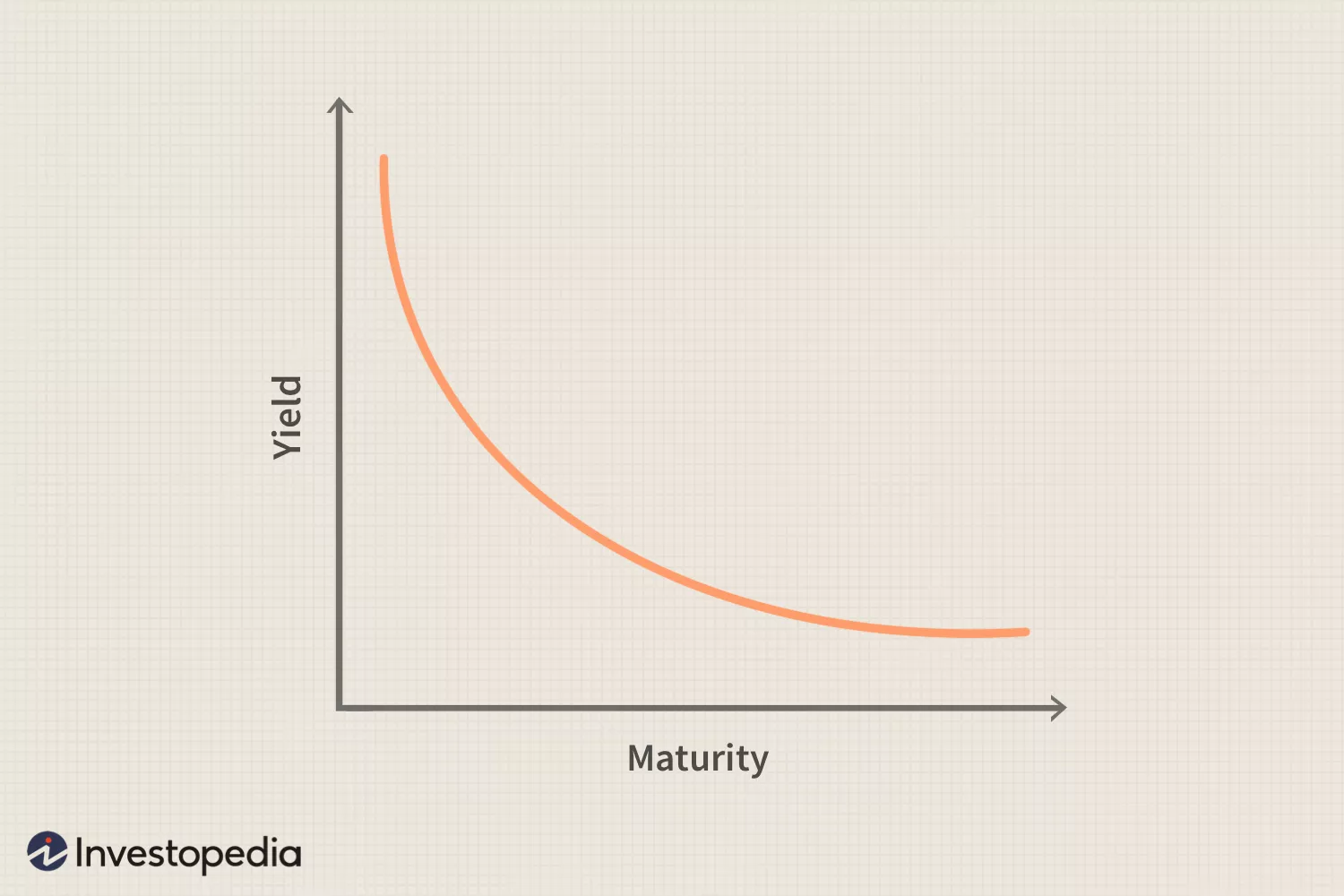Law of demand If a consumer’s demand for a good move in the same direction as the consumer’s income, the consumer’s demand for that good must be inversely related to the price of the good.

Law of diminishing marginal product, it states that advantages gained from slight improvement on the input side of the production equation will only advance marginally per unit and may level off or even decrease after a specific point.

Law of variable proportions The marginal product of a factor input initially rises with its employment level when the level of employment of the input is low. But after reaching a certain level of employment, it starts falling.

The long-run is a period of time in which all factors of production and costs are variable. In the long run, firms are able to adjust all costs, whereas, in the short run, firms are only able to influence prices through adjustments made to production levels.

Marginal cost Change is the total cost per unit of change in output.

Marginal product Change in output per unit of change in the input when all other inputs are held constant.

Marginal revenue (MR) is the increase in revenue that results from the sale of one additional unit of output. While marginal revenue can remain constant over a certain level of output, it follows from the law of diminishing returns and will eventually slow down as the output level increases. In economic theory, perfectly competitive firms continue producing output until marginal revenue equals marginal cost.

 The law of diminishing marginal returns is a theory in economics that predicts that after some optimal level of capacity is reached, adding an additional factor of production will actually result in smaller increases in output. For example, a factory employs workers to manufacture its products, and, at some point, the company operates at an optimal level. With all other production factors constant, adding additional workers beyond this optimal level will result in less efficient operations.

Marginal revenue product (MRP), also known as the marginal value product, is the marginal revenue created due to the addition of one unit of resource. The marginal revenue product is calculated by multiplying the marginal physical product (MPP) of the resource by the marginal revenue (MR) generated. The MRP assumes that the expenditures on other factors remain unchanged and helps determine the optimal level of a resource.

Marginal Cost of Production: The marginal cost of production is the change in total production cost that comes from making or producing one additional unit. To calculate marginal cost, divide the change in production costs by the change in quantity. The purpose of analyzing marginal cost is to determine at what point an organization can achieve economies of scale to optimize production and overall operations. If the marginal cost of producing one additional unit is lower than the per-unit price, the producer has the potential to gain a profit.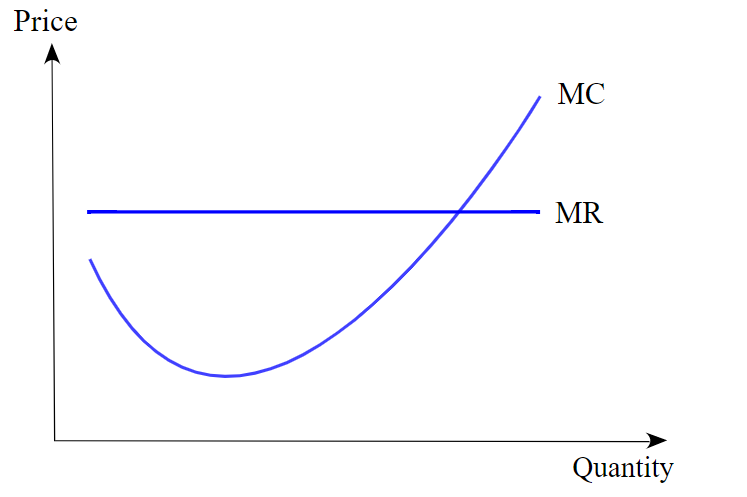The market supply curve shows the output levels that firms in the market produce in aggregate corresponding to different values of the market price.

Monopolistic competition is a market structure where there exists a very large number of sellers selling differentiated but substitutable products.

Monopoly A market structure in which there is a single seller and there are sufficient restrictions to prevent any other seller from entering the market.

Monotonic preferences A consumer’s preferences are monotonic if and only if between any two bundles, the consumer prefers the bundle which has more of at least one of the goods and no less of the other good as compared to the other bundle.

Normal good A good for which the demand increases with an increase in the income of the consumer is called a normal good.

Normal profit The profit level that is just enough to cover the explicit costs and opportunity costs of the firm is called the normal profit.

Oligopoly A market consisting of more than one (but few) sellers is called an oligopoly.

Opportunity costs represent the potential benefits an individual, investor, or business misses out on when choosing one alternative over another. The idea of opportunity costs is a major concept in economics.

Opportunity Cost Formula and Calculation

Opportunity Cost= FO−CO

• where:
• FO=Return on the best-foregone option.
• CO=Return on the chosen option.

Perfect competition A market environment wherein (i) all firms in the market produce the same good and (ii) buyers and sellers are price-takers.

A price ceiling is the mandated maximum amount a seller is allowed to charge for a product or service. Usually set by law, price ceilings are typically applied only to staples such as food and energy products when such goods become unaffordable to regular consumers. Some areas have rent ceilings to protect renters from rapidly climbing rates on residences.

Price elasticity of demand for a good is defined as the percentage change in demand for the good divided by the percentage change in its price.

Price elasticity of supply is the percentage change in quantity supplied due to a one percent change in the market price of the good.

Price floor The government-imposed lower limit on the price that may be charged for a particular good or service is called the price floor.

Price line is a horizontal straight line that shows the relationship between market price and a firm’s output level.

Price War, A price war is a competitive exchange among rival companies that lower the price points on their products, in a strategic attempt to undercut one another and capture greater market share. A price war may be used to increase revenue in the short term, or it may be employed as a longer-term strategy.

Productivity measures output per unit of input, such as labour, capital, or any other resource and is typically calculated for the economy as a whole, as a ratio of gross domestic product (GDP) to hours worked.

A production function shows the maximum quantity of output that can be produced by using different combinations of the inputs.

Profit is the difference between a firm’s total revenue and its total cost of production.

The short-run is a concept that states that, within a certain period in the future, at least one input is fixed while others are variable. It expresses the idea that an economy behaves differently depending on the length of time it has to react to certain stimuli. The short-run does not refer to a specific duration of time but rather is unique to the firm, industry, or economic variable being studied.

Shut down point is a level of operations at which a company experiences no benefit for continuing operations and therefore decides to shut down temporarily—or in some cases permanently. It results from the combination of output and price where the company earns just enough revenue to cover its total variable costs. The shutdown point denotes the exact moment when a company’s (marginal) revenue is equal to its variable (marginal) costs—in other words, it occurs when the marginal profit becomes negative.

 Marginal profit is the profit earned by a firm or individual when one additional or marginal unit is produced and sold. Marginal refers to the added cost or profit earned with producing the next unit. Marginal product is the additional revenue earned while the marginal cost is the added cost for producing one additional unit.

The substitution Effect The change in the optimal quantity of a good when its price changes and the consumer’s income is adjusted so that she can just buy the bundle that she was buying before the price change is called the substitution effect.

Super-normal profit Profit that a firm earns over and above the normal profit is called the super-normal profit.  Supernormal profit is also known as an abnormal profit. Abnormal profit means there is an incentive for other firms to enter the industry.

Total cost is the sum of total fixed cost and total variable cost.

Total fixed cost The cost that a firm incurs to employ fixed inputs is called the total fixed cost.

Total product If we vary a single input keeping all other inputs constant, then for different levels of employment of that input we get different levels of output from the production function. This relationship between the variable input and output is referred to as a total product.

Total return, when measuring performance, is the actual rate of return of an investment or a pool of investments over a given evaluation period. Total return includes interest, capital gains, dividends and distributions realized over a given period of time.

• Total return accounts for two categories of return: Income including interest paid by fixed-income investments, distributions or dividends, and capital appreciation, representing the change in the market price of an asset

Total revenue refers to the total receipts from sales of a given quantity of goods or services. It is the total income of a business and is calculated by multiplying the number of goods sold by the price of the goods.

Total revenue curve shows the relationship between a firm’s total revenue and the firm’s output level.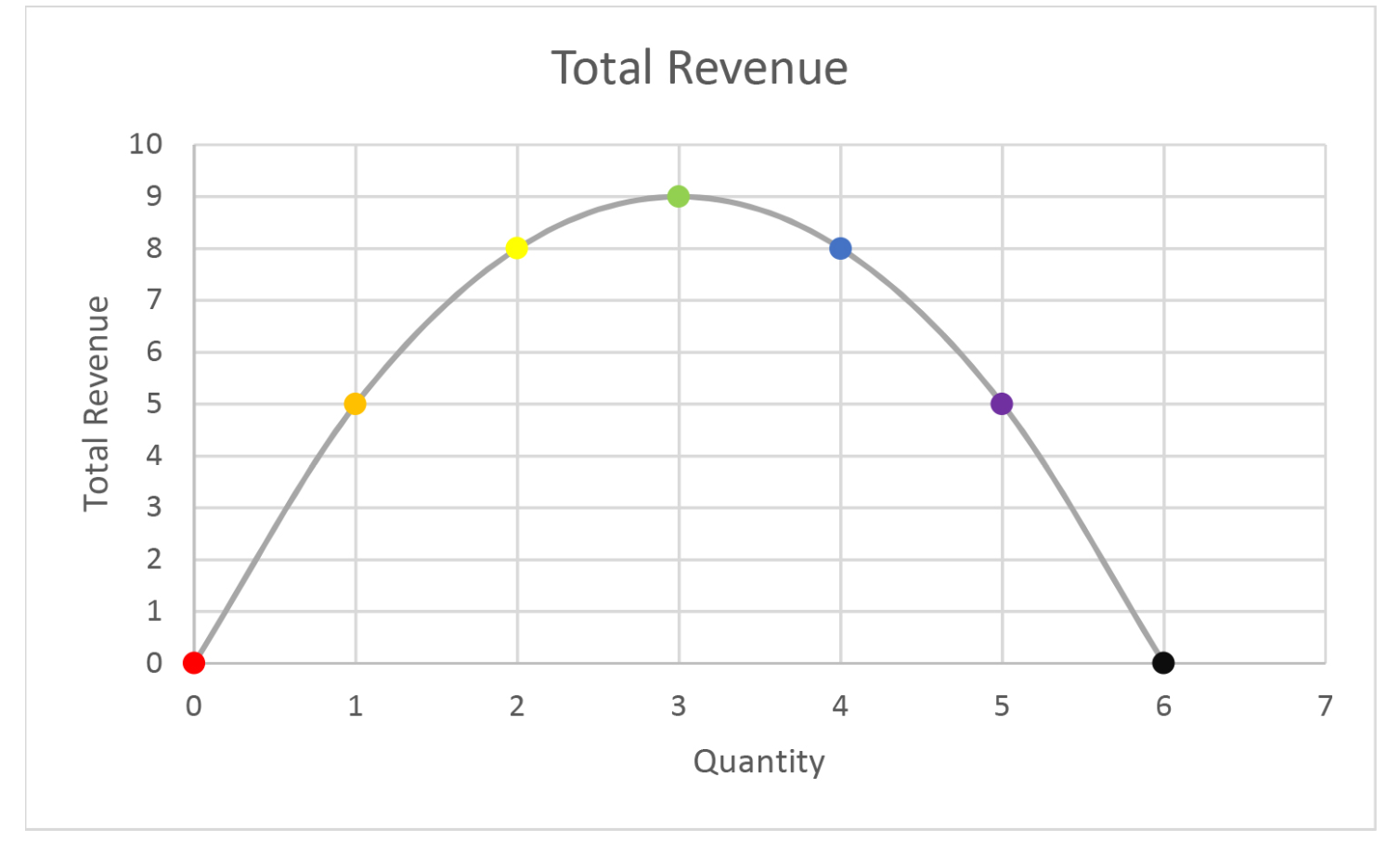Total variable cost The cost that a firm incurs to employ variable inputs is called the total variable cost.

Value of marginal product (VMP) is arrived at by multiplying the marginal physical product by the average revenue or the price of the product. The formula for calculating VMP is; Physical product × sales price of the product. VMP calculates the amount of a firm’s revenue that a unit of productive output contributes. VMP helps to prevent labour exploitation in industries.

Variable input An input the amount of which can be varied.

A Veblen good is a good for which demand increases as the price increases.

Please Share with maximum friends to support the Initiative.

### Enquire now

Give us a call or fill in the form below and we will contact you. We endeavor to answer all inquiries within 24 hours on business days.